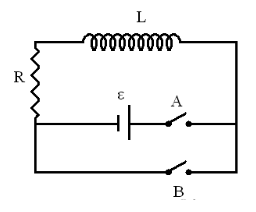# Problem: An RL circuit is shown on the right. L = 2.2 J, R = 258 Ω, ε = 4.5 VSwitch A is closed at t = 0. After a long time, when the current reaches its steady value, open switch A and close switch B at the same time. Count this moment as t = 0. Part (a) Calculate the numerical value of I at t = 0.1 s in amperes.Part (b) Calculate the numerical value of I at t = L/R s in amperes.Part (c) Calculate the current, in amperes, after an infinite time.

###### FREE Expert Solution

Current:

$\overline{){\mathbf{i}}{\mathbf{\left(}}{\mathbf{t}}{\mathbf{\right)}}{\mathbf{=}}{{\mathbf{i}}}_{{\mathbf{0}}}{{\mathbf{e}}}^{\mathbf{-}\frac{\mathbf{R}}{\mathbf{L}}\mathbf{t}}}$

Part (a)

i(0.1s) = i0e(-R/L)t = (ε/R)(e(-R/L)t) = (4.5/258)(e(-258/2.2)0.1) = 1.41 × 10-7 A

The current in the circuit at t = 0.1 s is 1.41 × 10-7 A###### Problem Details

An RL circuit is shown on the right. L = 2.2 J, R = 258 Ω, ε = 4.5 VSwitch A is closed at t = 0.

After a long time, when the current reaches its steady value, open switch A and close switch B at the same time. Count this moment as t = 0.

Part (a) Calculate the numerical value of I at t = 0.1 s in amperes.

Part (b) Calculate the numerical value of I at t = L/R s in amperes.

Part (c) Calculate the current, in amperes, after an infinite time.